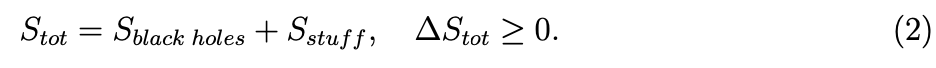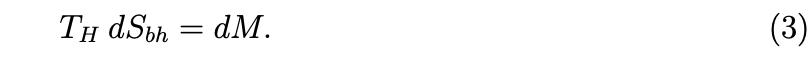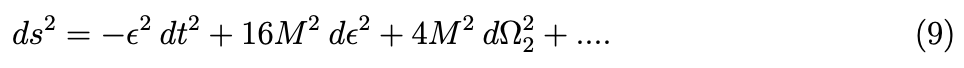top of page
Search

# A Poor Woman’s Derivation of a Covariant Entropy Bound: Part One

Laura Johnson, PhD

ETH ZurichAlthough this two part blog is geared towards our readers with a bit of a physics background, part one is intended to be accessible to a wide audience. For some background on related topics in this post, we have a few podcasts and blog posts for you to check out. For some black hole basics, check out our first podcast Black Holes are Everywhere. We also have a podcast that explains entropy alongside black holes with some introductory examples called Entropy, Black Holes, and the Heat Death of the Universe. Additionally, we will refer you to related blog posts throughout this work when we think it might be useful (for some basic motivation for fundamental physics check out this blog).

In today’s blog, we introduce black hole entropy and the tools needed to calculate it. We will be focusing on the Bekenstien entropy bound which puts an upper bound on the entropy needed to describe physical systems, like a cup of tea or a globular cluster. Using a Gedanken experiment, we derive the Bekenstein entropy bound. Take note that this bound is only satisfied for systems of constant, finite size, and weak self-gravity. What is weak self-gravity? For now, weak self-gravity can be loosely motivated by thinking of situations in which the effect of gravity isn't too strong.

The latter half of this blog (to be released at a future date) will get more technical. In part two, the Bekenstein bound will be reformulated into a covariant form, known as the covariant entropy bound, proposed by Bousso, which is valid in all space-times admitted by Einstein’s equation. Stay tuned for the release of part two which will be on our blog and linked here.

## IntroductionEntropy can be thought of as counting the number of microstates that a system can be in. For example, let's consider flipping a coin with two sides, heads and and tails. In our example, we will flip the coin 4 times. The microstates of this example would be the possible outcomes: HHHH, HHHT, ... , TTTT.If we choose to measure the number of tails, this could be our macrostate. The entropy of a given macrostate would correspond to the logarithm of the number of microstates that corresponds to it. A macrostate with lower entropy would have fewer microstates than a macrostate with higher entropy. So a macrostate with 4 tails has one microstate and an entropy of S=log(1)=0, which a macrostate with 2 tails has 6 microstates and and entropy of S=log(6) ~ 1.79. And we can count quantum microstates too (for a blog post about some modern debates in quantum mechanics see Bardeen’s Ass by Christian Jepsen). In physics, the Bekenstein bound puts an upper limit on the amount of entropy S, or information that can be contained in a finite region of space with a finite amount of energy. In other words, it puts constraints on the maximum amount of information needed to exactly describe the system down to the quantum level.Yet, entropy bounds have implications even outside the realm of physics. In computer science, this implies that a Turing machine with finite size cannot have an unbounded memory. There is a maximum information-processing rate. Even in theory, the human brain cannot hold infinite amounts of information. An average human brain of mass 1.5 kg and volume 1250 cm^3 has an information Bekenstein bound of ≈ 2.6x10^42 bits*. This post will give an informal derivation of the Bekenstein bound using a thought experiment. There are more rigorous Quantum Field Theory derivations, but to keep within the spirit of general relativity and how relativity was first invented using thought experiments, we still stick to these informal sorts of arguments for now.

In part two of this post, we will show how this bound cannot be the full story as it is not covariant and does not hold when the conditions of finite size, energy, and weak self-gravity are not met. It will be succeeded by a covariant version known as the Bousso bound. Nevertheless, the Bousso bound reduces to the case of the Bekenstein bound for the case of weak self-gravity and finite size/energy. It has also been shown to be valid for many difference cases including surfaces inside gravitationally collapsing objects and cosmological solutions to Einstein’s equation  (see The Cosmological Constant Problem by Leah Jenks for an introduction to the using Einstein’s equations in cosmology).

## Black Hole Entropy

In order to talk about entropy bounds, it is beneficial to first understand black hole entropy. Black holes arise as classical solutions to Einstein’s theory of general relativity and can be completely characterized, according the no hair theorem, by only three parameters: mass, electric charge, and angular momentum. Since they can be characterized by just these three parameters, it is perplexing that they should even have entropy. Entropy, S, in statistical mechanics counts the number of microstates of a system:However, classically, black holes have no microstates. It seems that black holes would have no entropy as they do not have any microstates. On the other hand, if we had a cup of tea with some amount of entropy, and we threw it into the black hole, we would end up with a black hole with a slightly larger mass. If black holes did not have entropy, the entropy of the tea would have disappeared violating the second law of thermodynamics. One could even imagine using black holes as an entropy dumping ground to create a perpetual motion machine as depicted in Figure 1, where a black hole is used to convert gravitational potential energy into mechanical energy, which is then turned into electrical energy to power a light bulb. In this drawing, a box of thermal radiation is lowered to just outside the black hole horizon. The radiation is then dumped onto the black hole horizon, and the box is lifted back up using less work than was generated during the lowering process. The box can then be refilled from the reservoir and the process repeated. To be consistent with the second law, black holes must have entropy and if we throw a cup of tea into a black hole, the change in the black hole entropy must be greater than or equal to the lost entropy of the tea :Now that we see the motivation for needing black holes to have entropy, we can go about figuring out how to calculate the entropy of a black hole.

The image below was conceived of by Robert Geroch, drawn by Louis Fulgoni, and was copied from Bekenstein (1980).Gravitational thermodynamic engine. 

## Calculating Black Hole Entropy

For simplicity, we will stick with Schwarzchild black holes, which can be characterized by a mass M. From the first law of thermodynamics, dE = TdS, where E is energy, T is temperature. Classically, black holes have a temperature of absolute 0, but when quantum effects are taken into account, black holes behave as perfect blackbodies, absorbing and emitting particles (Hawking radiation) at a temperature know as the Hawking temperature T_H. Using E = M we find [3–5].The Hawking temperature can be found using a periodicity trick. To perform this trick, two facts must first be proven.

Fact 1: At finite temperature, quantum field theory is periodic in imaginary time.This can be shown using the thermal Green’s function defined as (4)where O is an operator, τ = it is Euclidean time, and T_E mean Euclidean time ordering.

This Green’s function is periodic in imaginary time:where in the 1st line we assume 0 < τ < β, in the 2nd line we use the cyclic property of the trace, in the 3rd line we use the definition of time translation:Thus t ∼ t + iβ [3, 5].

Fact 2: The region near the horizon of black holes can be related to Rindler space.While this holds for other black holes as well, for our purposes, showing it holds for a Schwarzchild black hole will suffice. The metric for the Schwarzchild black hole is:To look at the near horizon region, we can make the coordinate changewhere ε≪1. Expanding about small ε we getNow, make another coordinate change:to see that we get the Rindler form in the (R, η) piece:The horizon is at R = 0, so we restrict the coordinates to R > 0, but there is no restriction on η. Now, if we make one last transformation, η = iφ, then we see that we get something that looks like polar coordinates in the (R, η) piece:with the identificationFinally, we conclude that β = 8πM, so the Hawking temperature of a Schwarzchild black hole isPlugging this back into equation (3), we getIntegrating this and assuming Sbh → 0 as M → 0, we getor putting back in factors of G, h bar, c,Using the entropy of a Schwarzchild black hole, we can now derive an entropy bound.

## Bekenstein Bound

The necessity of a maximum entropy bound for an object or system with a given size R and energy E can be realized by a simple Gedanken experiment. This is known as the “poor man’s derivation of the Bekenstein bound,” as a more rigorous derivation can be done using quantum field theory. Starting with a Schwarzchild black hole of mass M and entropy Sbh = 4π(G/hbar c)M^2, imagine adiabatically dropping a cup of tea of mass m ≪ M and entropy S from far away. After sipping the cup oftea, the black hole mass will grow to M + m, assuming energy losses to gravitational radiation and Hawking radiation are negligible. So the entropy of the black hole will beThe black hole’s entropy grows by an amount ∆Sbh = 8π(G/hbar c)(Mm) to first order in m, while the cup of tea along with it’s entropy has disappeared. In order to obey the second law of thermodynamics, S + 8π(G/hbar c)(Mm) ≥ 0. This can be rewritten in terms of the radius of the black hole horizon, rh = 2GM/c^2 to getUniversal Bounds 

We now take the limit that the black hole radius is as small as we can make it, maybe a few times bigger than the radius cup of tea, to ensure the cup of tea can still be swallowed by the black hole, rh = ξR, where ξ is some number larger than, but on the order of unity. Our condition that m ≪ M is still satisfied because we assume the cup of tea to be much larger than its gravitational radius 2GM/c . Using E = mc , we can write the entropy bound as. There are more formal approaches that can be used to derive this, and it can be found that ξ = 1/2. To give an idea of where the entropy of various objects lie in relation to their Bekenstein bound, Figure 2 is a plot of the entropy bound versus the radius of systems with various densities. The equation for this plot can be obtained starting with the Bekenstein bound equation, plugging in E = mc^2, and using the Wigner-Seitz radius, R = (3m/4πρ)^1/3 to write mass m in terms of density ρ and size R:The figure is over-plotted with the entropy and size of various objects to compare to the bounds; the neutron star should be compared with the purple line bound, the globular cluster with the green line bound, and the rest against the blue line bound .

That concludes the first portion of this post! We went over some great foundational concepts about entropy and black holes that will serve us well for part two where we get to the heart of this poor woman's derivation of a covariant entropy bound.

There were many subtleties that were glossed over during the derivations in this paper in order to keep within the theme of derivation by thought experiments. In summary, as Bousso puts it ,

## “Information tells spacetime how to curve; spacetime tells information how to disappear.”

 Wikipedia. Bekenstein bound — wikipedia, the free encyclopedia, 2016. [Online; accessed29-April-2016]. Black Hole Event Horizon Image

 J. D. Bekenstein. Bekenstein bound. 3(10):7374, 2008. revision 121148. Image for gravitational-thermodynamic engine was conceived of by Robert Geroch, drawn by Louis Fulgoni, and was copied from Bekenstein (1980). Image for the Universal Bounds Plot can be found here on scholarpedia.

 Tom Hartman.Quantum Gravity and Black Holes, Spring 2015.

 Barton Zwiebach.A First Course in String Theory. Cambridge University Press, 2004.

 Robert Wald.General Relativity. The University of Chicago Press, 1984.

 Charles W Misner, Kip S Thorne, and John Archibald Wheeler.Gravitation. W H Freemanand Company, 1973.

 Rafael Bousso. Perturbative proof of the covariant entropy bound. FXQI Conference in Vieques,2014.

 Raphael Bousso, Horacio Casini, Zachary Fisher, and Juan Maldacena. Proof of a quantumbousso bound.Physical Review D, 90(4), Aug 2014.

 Raphael Bousso. A covariant entropy conjecture.Journal of High Energy Physics, 1999(07):004,1999.

*Note that there are different ways to define entropy. While in the table for coin flipping log base 10 was used, here it is log base 2.

See All
bottom of page